# 62. Unique Paths I & II

Reference: Problem I & Problem II
Difficulty: Medium

My Post:

## Problem

A robot is located at the top-left corner of a m x n grid (marked ‘Start’ in the diagram below).

The robot can only move either down or right at any point in time. The robot is trying to reach the bottom-right corner of the grid (marked ‘Finish’ in the diagram below).

How many possible unique paths are there?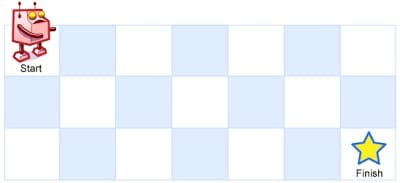Note: m and n will be at most 100.

Example:

## Analysis

### Recursion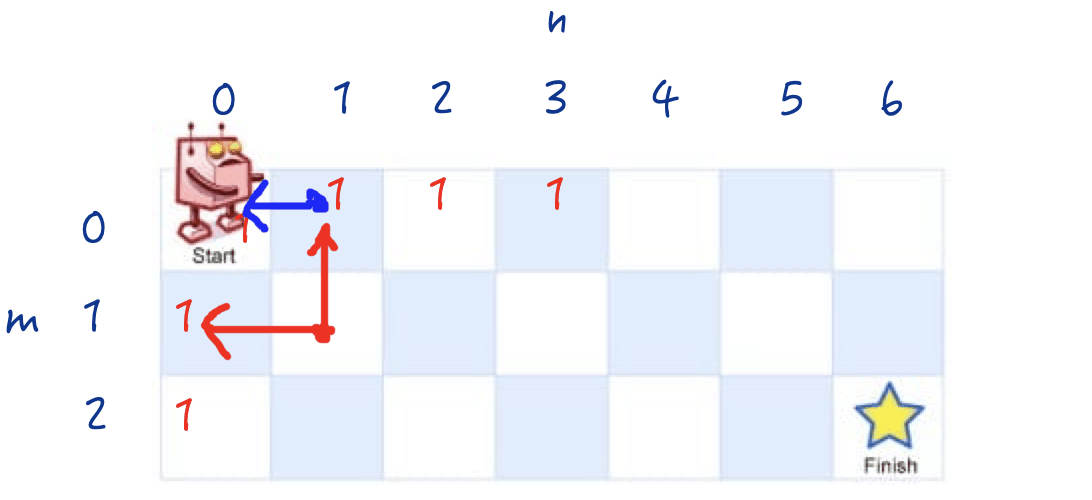Time: $O(2^{M + N})$
Space: $O(M + N)$

Recurrence Tree for complexity analysis: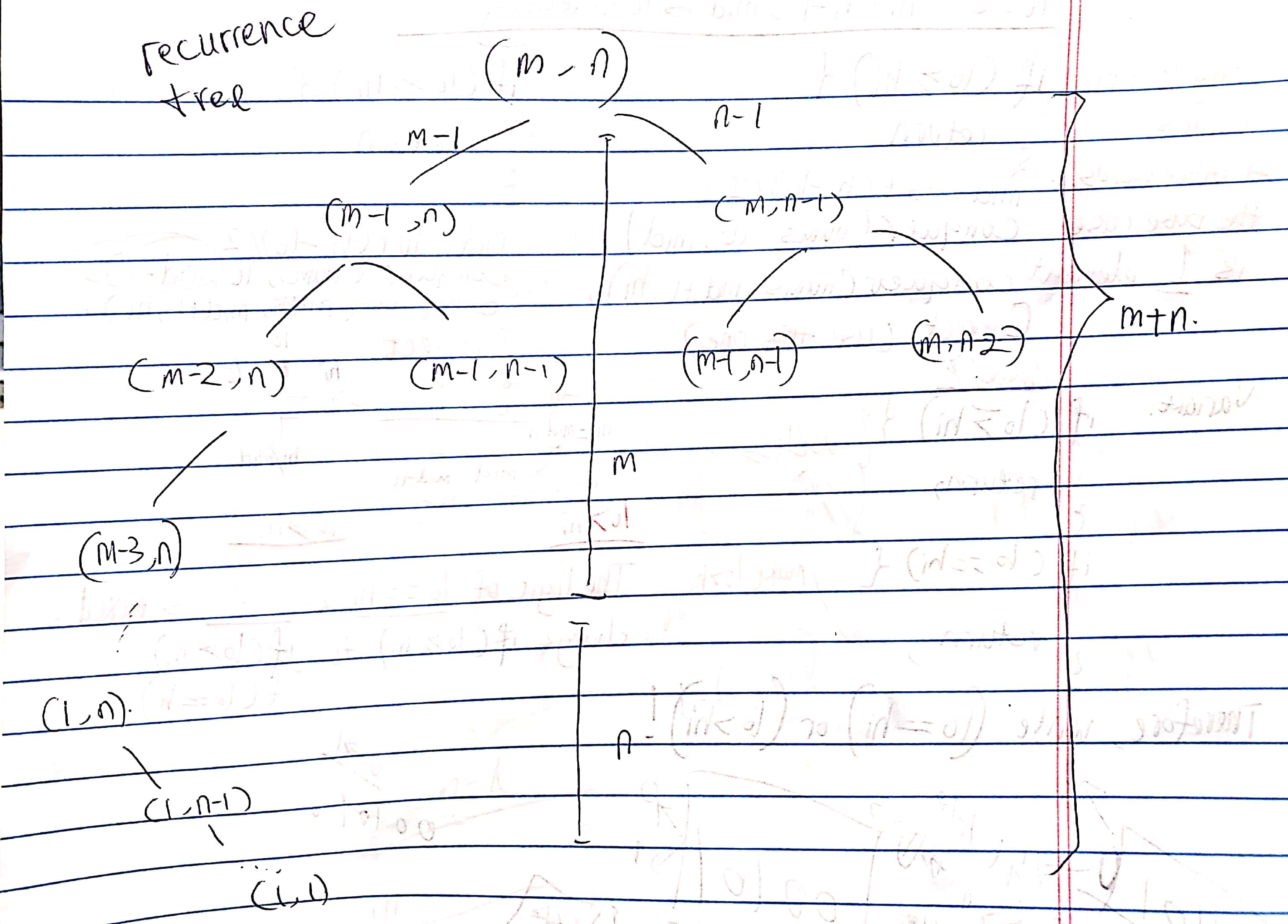### DP (Top-down with Memoization)

Use an 2D array mem to do memoization.

Time: $O(MN)$ where $MN$ is the number of subproblems.
Space: $O(MN)$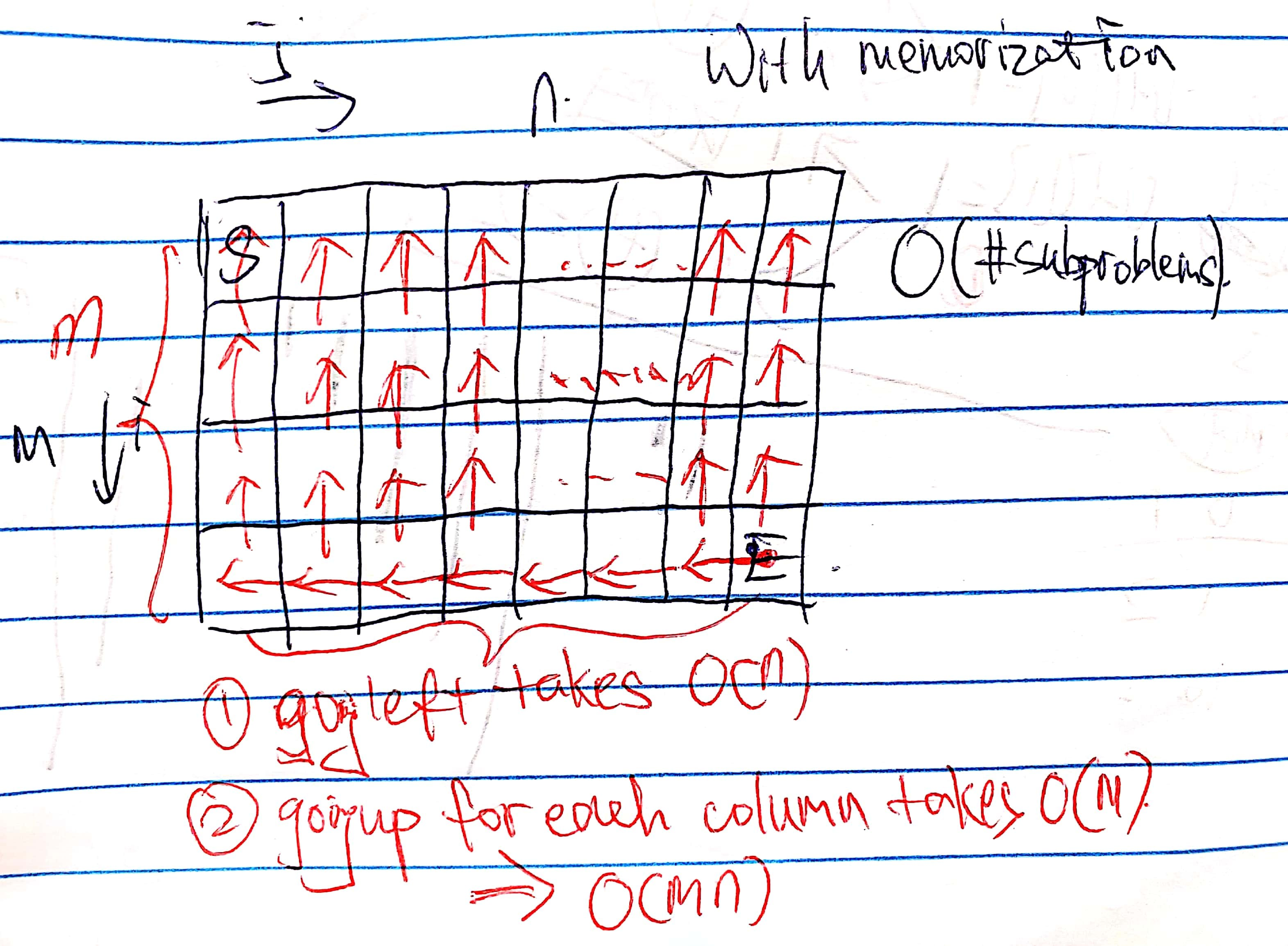### DP (Bottom-up)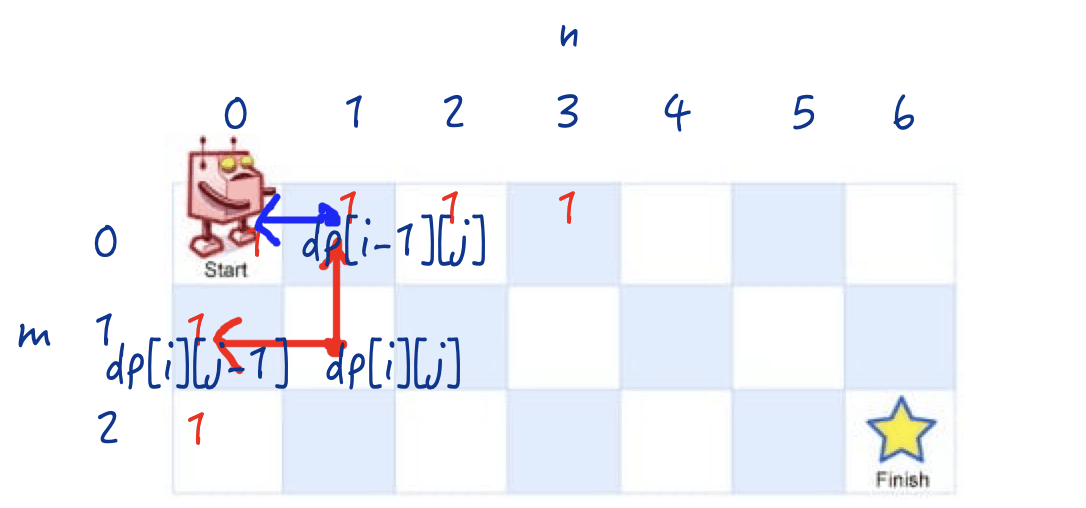Time: $O(MN)$
Space: $O(MN)$

### DP (Bottom-up, Linear Space)

Reduce the $O(MN)$ space complexity to $O(N)$ (a row) or $O(M)$ (a column). In terms of a row, we would update dp[j] by its old value plus dp[j - 1].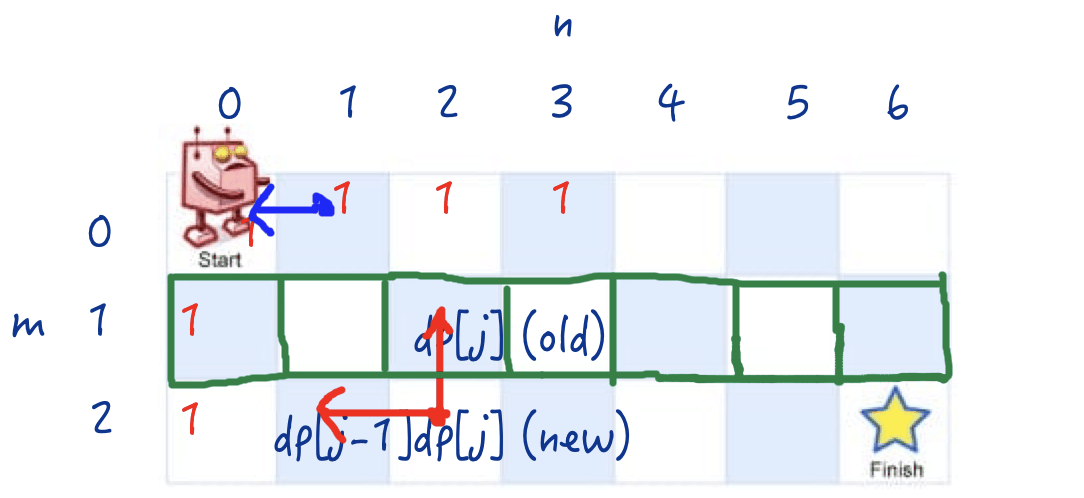Time: $O(MN)$
Space: $O(N)$ or $O(M)$

## Unique Paths II (with Obstacles)

Now consider if some obstacles are added to the grids. How many unique paths would there be?

An obstacle and empty space is marked as 1 and 0 respectively in the grid.

Example:

### DP (Bottom-up)

The problem is very great. Check out the picture below. Here are some points to be considered carefully:

• What two surrounding cells are affected if a cell is blocked?
• Do we need to change the recurrence in the code?
• No. If blocked, just setting dp[i][j] as $0$ is all we need.
• What happens if the starting point or the finish point is blocked?
• In the initial step, what happens to its following cells if a cell is blocked?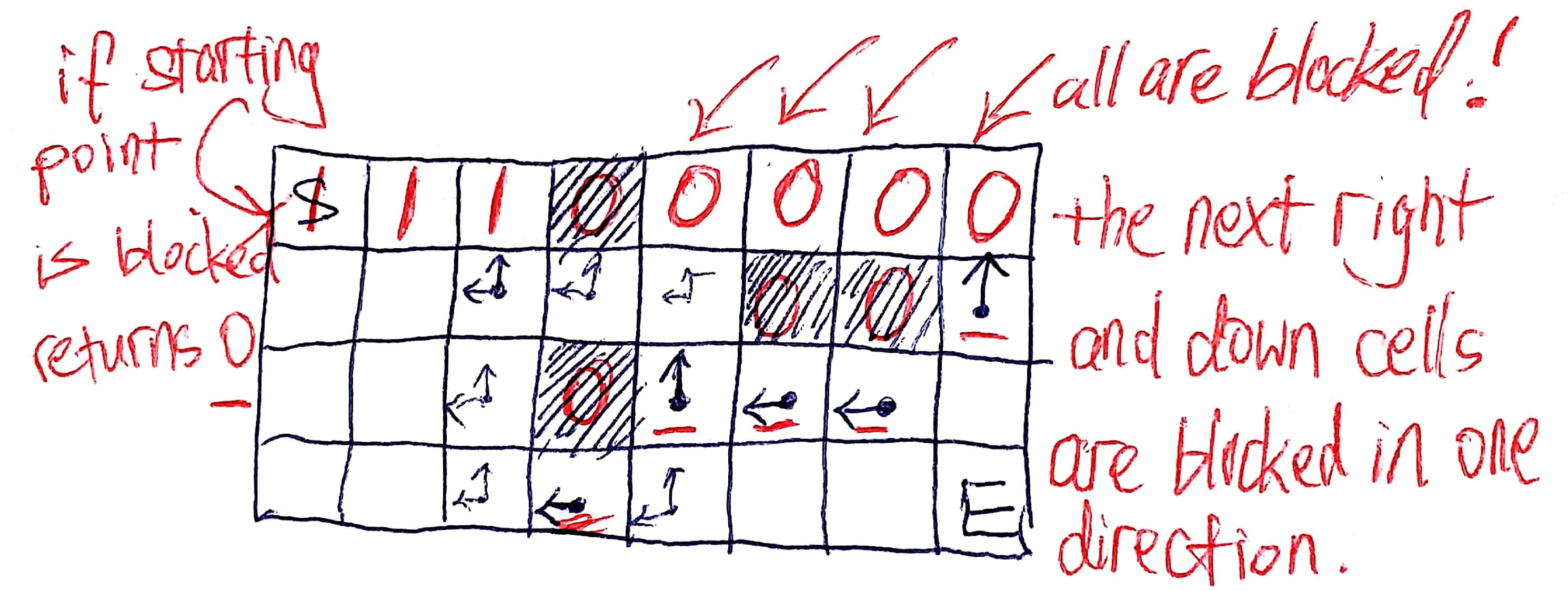Time: $O(MN)$
Space: $O(MN)$

### DP (Bottom-up, Linear Space)

We can reduce the space complexity too, but be careful when actually writing the code! In the row case, we need check if a cell at the first column is blocked in the dp step every time, which is usually forgotten.

Time: $O(MN)$
Space: $O(N)$ or $O(M)$

CommentJunhao Wang
a software engineering cat